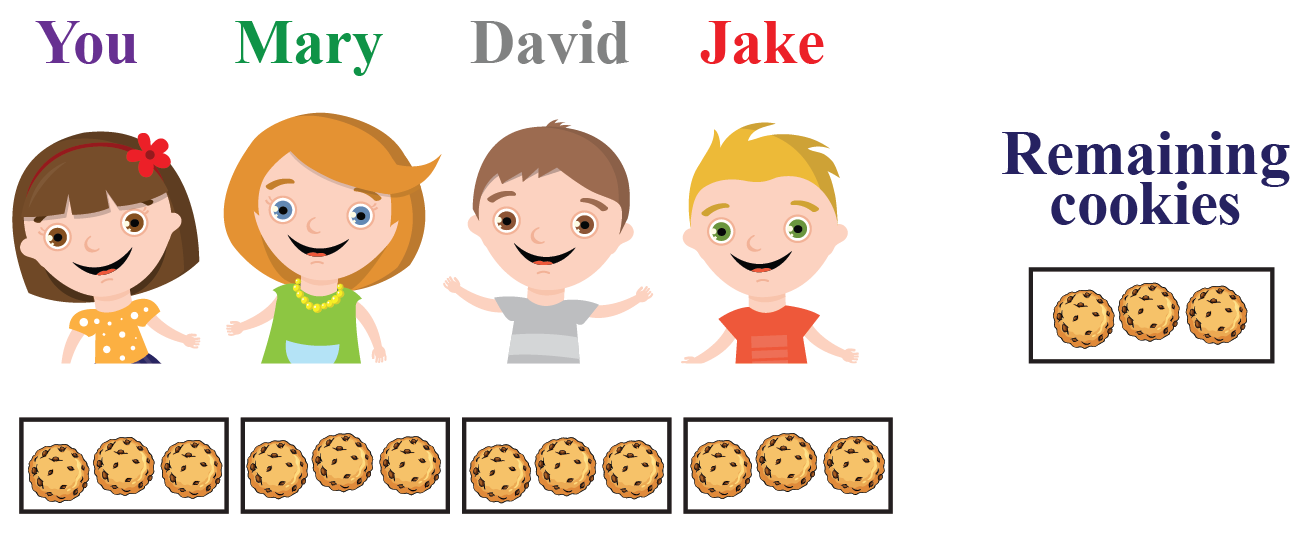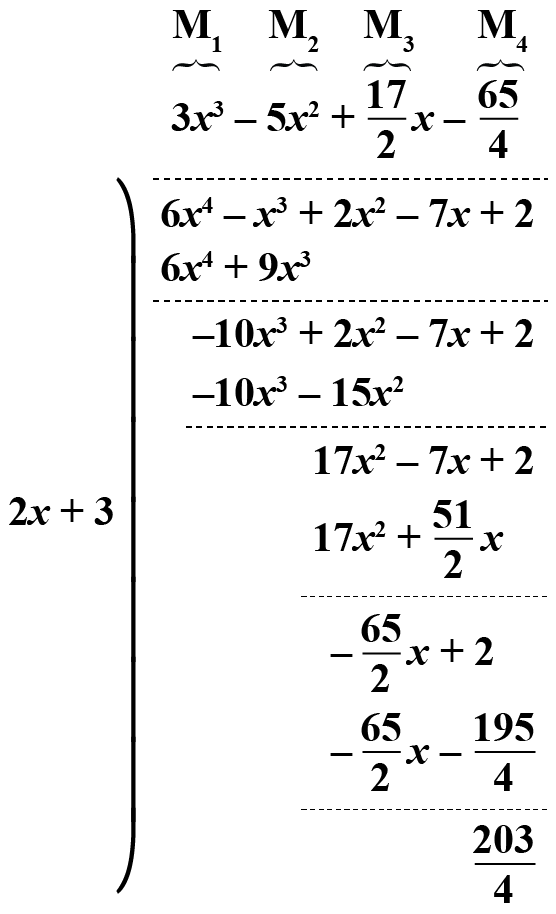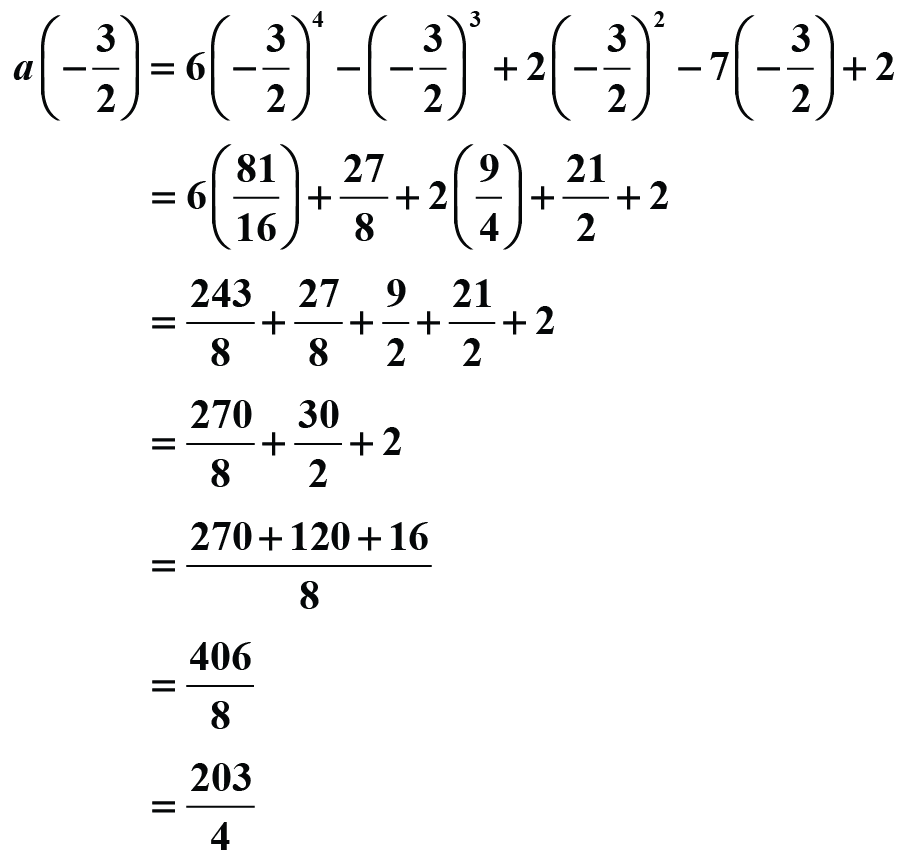# Remainder Theorem

When a certain number of things are divided into groups with an equal number of things in each group, the number of leftover things is known as the remainder.

It is something that "remains" after division.

Let us consider an example.

Assume that you have 15 cookies that you want to share with 3 of your friends, Mary, David, and Jake.

You want to share the cookies equally among your friends and yourself.

You begin distributing them in the following way (shown in the image).

Here, you can see that there are 3 cookies remaining after the distribution.

These 3 cookies cannot be further shared equally among the 4 of you.

Hence, 3 is called the remainder here.With this, we learned about the basics of the remainder. Learning the concept of the remainder theorem will now come easily to us.

In this mini-lesson, we explore the world of the remainder theorem. We walk through answers to questions like what is remainder theorem, formula of remainder theorem, and how does remainder theorem work, along with solved examples and interactive questions.

## Lesson Plan

 1 What is the Remainder Theorem? 2 Important Notes 3 Solved Examples 4 Interactive Questions 5 Challenging Question

## What is the Remainder Theorem?

The remainder theorem is stated as follows: When a polynomial a(x) is divided by a linear polynomial b(x) whose zero is x = k, the remainder is given by r = a(k).

The remainder theorem enables us to calculate the remainder of the division of any polynomial by a linear polynomial, without actually carrying out the steps of the division algorithm.

## What Is Remainder Theorem Formula?

### Remainder Theorem Formula

The general formula for remainder theorem is

 p(x) = (x-c)·q(x) + r(x)

Let us consider polynomials to prove the remainder theorem formula.

You know that Dividend = (Divisor × Quotient) + Remainder

If r(x) is the constant then, p(x) = (x-c)·q(x) + r

Let us put x=c

p(c) = (c-c)·q(c) + r

p(c) = (0)·q(c) + r

p(c) = r

Hence, proved.

## How Does Remainder Theorem Work?

To understand how the remainder theorem works, let us consider a general case. Let a(x) be the dividend polynomial and b(x) the linear divisor polynomial, and let q(x) be the quotient and r the constant remainder. Thus, we have

a(x) = b(x) q(x) + r

Let us denote the zero of the linear polynomial b(x) by k. This means that

b(k) = 0

If we plug in x as k in the starred relation above, we have

a(k) = b(k) q(k) + r

Note that doing this is allowed since the starred relation holds true for every value of x. In fact, it is a polynomial identity. Since b(k)=0 we are left with a(k)=r.

In other words, the remainder is equal to the value of a(x) when x is equal to k. Precisely what we stumbled upon!

This is exactly what the remainder theorem is: When a polynomial a(x) is divided by a linear polynomial b(x) whose zero is x equal to k, the remainder is given by r=a(k).

To see how it works in the case of polynomials, let us consider the following example with two polynomials:

a(x) : 6x4 - x3 + 2x2 - 7x + 2
b(x) : 2x + 3On dividing polynomials, the quotient polynomial and the remainder are:

q(x) = 3x3 - 5x2 + 17/2 x - 65/4 r = 203/4

We calculated the remainder in this case to be r = 203/4. Now, let’s see what happens when we evaluate a(x) for x equal to the zero of b(x), which is x = -3/2. We haveOnce again, this has turned out to be equal to the remainder we calculated using the division algorithm.

Polynomials
Grade 9 | Questions Set 1
Polynomials
Polynomials